Next: 4. Cost-Sensitive Modeling Up: Toward Cost-Sensitive Modeling for Previous: 2. Cost Factors and

# 3. Cost Models

A cost model formulates the total expected cost of intrusion detection. It considers the trade-off among all relevant cost factors and provides the basis for making appropriate cost-sensitive detection decisions. We first examine the cost trade-off associated with each possible outcome of observing some event e, which may represent a network connection, a user's session on a system, or some logical grouping of activities being monitored. In our discussion, we say that e=(a, p, r) is an event described by the attack type a (which can be normal for a truly normal event), the progress p of the attack, and the target resource r. The detection outcome of e is one of the following: false negative (FN), false positive (FP), true positive (TP), true negative (TN), or misclassified hit. The costs associated with these outcomes are known as consequential costs (CCost), as they are incurred as a consequence of prediction, and are outlined in Table 2.

FN Cost is the cost of not detecting an attack and is incurred by systems that do not install IDSs. Here, the IDS falsely decides that a connection is not an attack and does not respond to the attack. This indicates that the attack will succeed and the target resource will be damaged. The FN Cost is therefore defined as the damage cost associated with event e, or DCost(e).

TP Cost is incurred in the event of a correctly classified attack, and involves the cost of detecting the attack and possibly responding to it. To determine whether response will be taken, RCost and DCost must be considered. If the damage done by the attack to resource r is less than RCost, then ignoring the attack actually reduces the overall cost. Therefore, if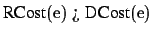, the intrusion is not responded to beyond simply logging its occurrence, and the loss is DCost(e). If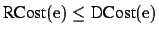, then the intrusion is acted upon and the loss is limited to RCost(e). In reality, however, by the time an attack is detected and response ensues, some damage may have incurred. To account for this, TP cost may be defined as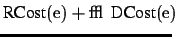, where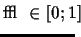is a function of the progress p of the attack.

FP Cost is incurred when an event is incorrectly classified as an attack, i.e., when e=(normal,p,r) is misidentified as e'=(a, p', r) for some attack a. If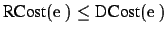, a response will ensue and the response cost, RCost(e'), must be accounted for as well. Also, since normal activities may be disrupted due to unnecessary response, false alarms should be penalized. For our discussion, we use PCost(e) to represent the penalty cost of treating a legitimate event e as an intrusion. For example, if e is aborted, PCost(e) can be the damage cost of a DOS attack on resource r, because a legitimate user may be denied access to r.

TN Cost is always 0, as it is incurred when an IDS correctly decides that an event is normal. We therefore bare no cost that is dependent on the outcome of the decision.

Misclassified Hit Cost is incurred when the wrong type of attack is identified, i.e., an event e=(a, p, r) is misidentified as e'=(a', p', r). If, a response will ensue and RCost(e') needs to be accounted for. Since the response taken is effective against attack type a'rather than a, some damage cost of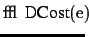will be incurred due to the true attack. Hereis a function of the progress p and the effect of the response intended for a' on a.

We can now define the cost model for an IDS. When evaluating an IDS over some labeled test set E, where each event,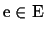, has a label of normal or one of the intrusions, we define the cumulative cost of the IDS as follows: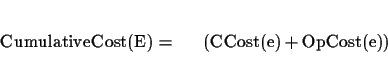(1)

where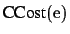, the consequential cost of the prediction by the IDS on e, is defined in Table 2.

 Outcome Consequential Cost CCost(e) Condition Miss (False Negative, FN) DCost(e) False Alarm (False Positive, FP)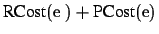if DCost(e')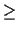RCost(e') or 0 if DCost(e') < RCost(e') Hit (True Positive, TP)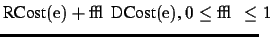if DCost(e)RCost(e) or DCost(e) if DCost(e) < RCost(e) Normal (True Negative, TN) 0 Misclassified Hit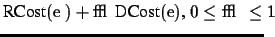if DCost(e')RCost(e') or DCost(e) if DCost(e') < RCost(e')

It may not always be possible to fold damage and response costs into the same measurement unit. Instead, each should be analyzed in its own relative scale. We must, however, compare and then combine the two so that we can compute CCost(e) for use in the calculation of CumulativeCost in Equation 1. One way is to decide first under what conditions to not respond to particular intrusions. For example, assuming that probing attacks should not be responded to and that the damage cost for probing is 2, then the response cost for probing must be greater, say, 20. Similarly, if the attack type with the lowest damage cost should not be ignored, then the corresponding lowest response cost should be a smaller value. Once a starting value is defined, remaining values can be computed according to the relative scales discussed in Section 2.2.

OpCost(e) in Equation 1 can be computed as the sum of the computational costs of all the features used during rule checking. Since OpCost(e) and CCost(e) use two different measurement units and there is no possibility of comparing the two, as with damage cost and response cost, we can use Equation 1 at a conceptual level. That is, when evaluating IDSs, we can consider both the cumulative OpCost and cumulative CCost, but actual comparisons are performed separately using the two costs. This inconvenience can not be overcome easily unless all cost factors can be represented using a common measurement unit, or there is a reference or comparison relation for all the factors. Site-specific policies can be used to determine how to uniformly measure these factors.Next: 4. Cost-Sensitive Modeling Up: Toward Cost-Sensitive Modeling for Previous: 2. Cost Factors and